## Fourier Decomposition of Radiation Oscillators

Our goal is to write the Hamiltonian for the radiation field in terms of a sum of harmonic oscillator Hamiltonians. The first step is to write the radiation field in as simple a way as possible, as a sum of harmonic components. We will work in a cubic volumeand apply periodic boundary conditions on our electromagnetic waves. We also assume for now that there are no sources inside the region so that we can make a gauge transformation to makeand hence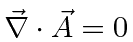. We decompose the field into its Fourier components atwhere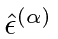are real unit vectors, andis the coefficient of the wave with wave vectorand polarization vector. Once the wave vector is chosen, the two polarization vectors must be picked so that,, andform a right handed orthogonal system. The components of the wave vector must satisfydue to the periodic boundary conditions. The factor out front is set to normalize the states nicely sinceandWe know the time dependence of the waves from Maxwell's equation,where. We can now write the vector potential as a function of position and time.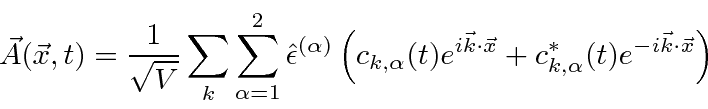We may write this solution in several different ways, and use the best one for the calculation being performed. One nice way to write this is in terms 4-vector, the wave number,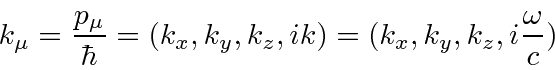so thatWe can then write the radiation field in a more covariant way.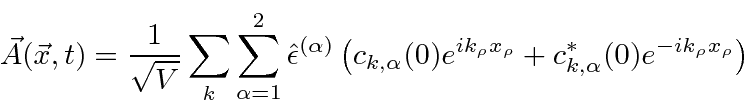A convenient shorthand for calculations is possible by noticing that the second term is just the complex conjugate of the first.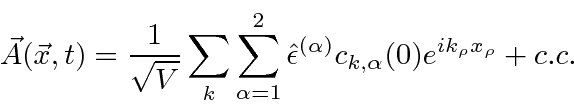Note again that we have made this a transverse field by construction. The unit vectorsare transverse to the direction of propagation. Also note that we are working in a gauge with, so this can also represent the 4-vector form of the potential. The Fourier decomposition of the radiation field can be written very simply.This choice of gauge makes switching between 4-vector and 3-vector expressions for the potential trivial.

Let's verify that this decomposition of the radiation field satisfies the Maxwell equation, just for some practice. Its most convenient to use the covariant form of the equation and field.The result is zero since.

Let's also verify that.The result here is zero because.

Jim Branson 2013-04-22